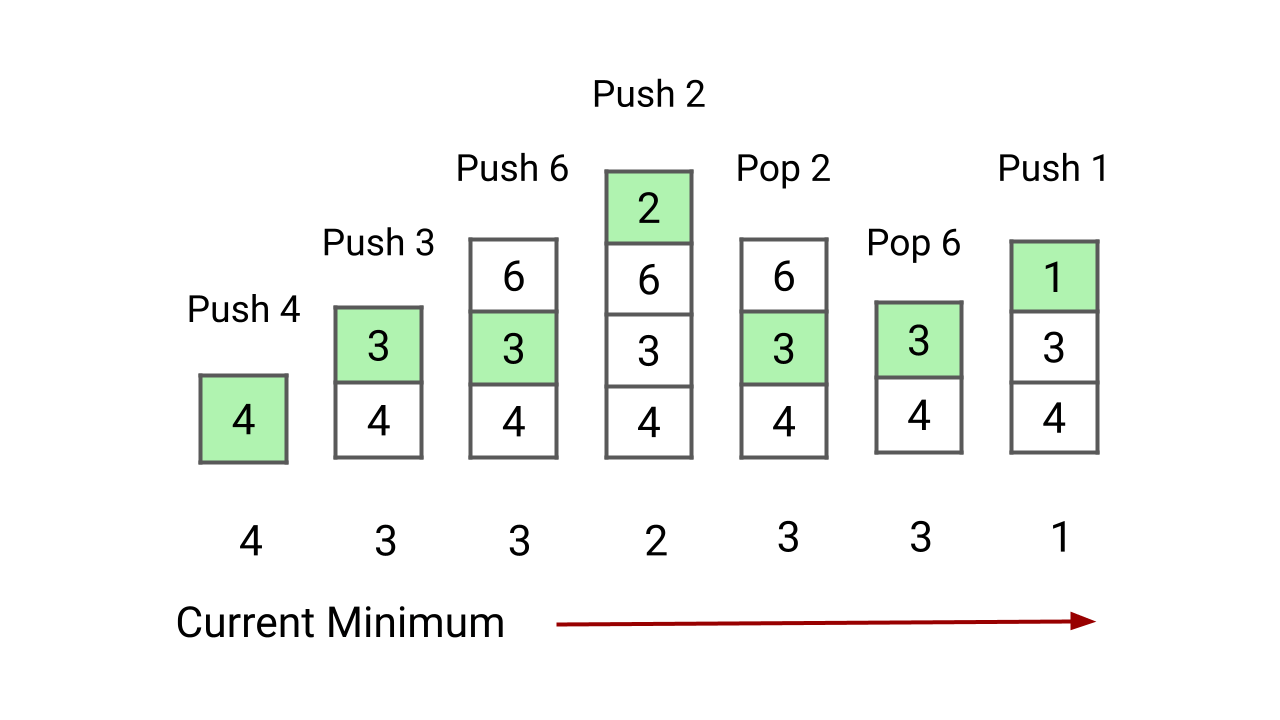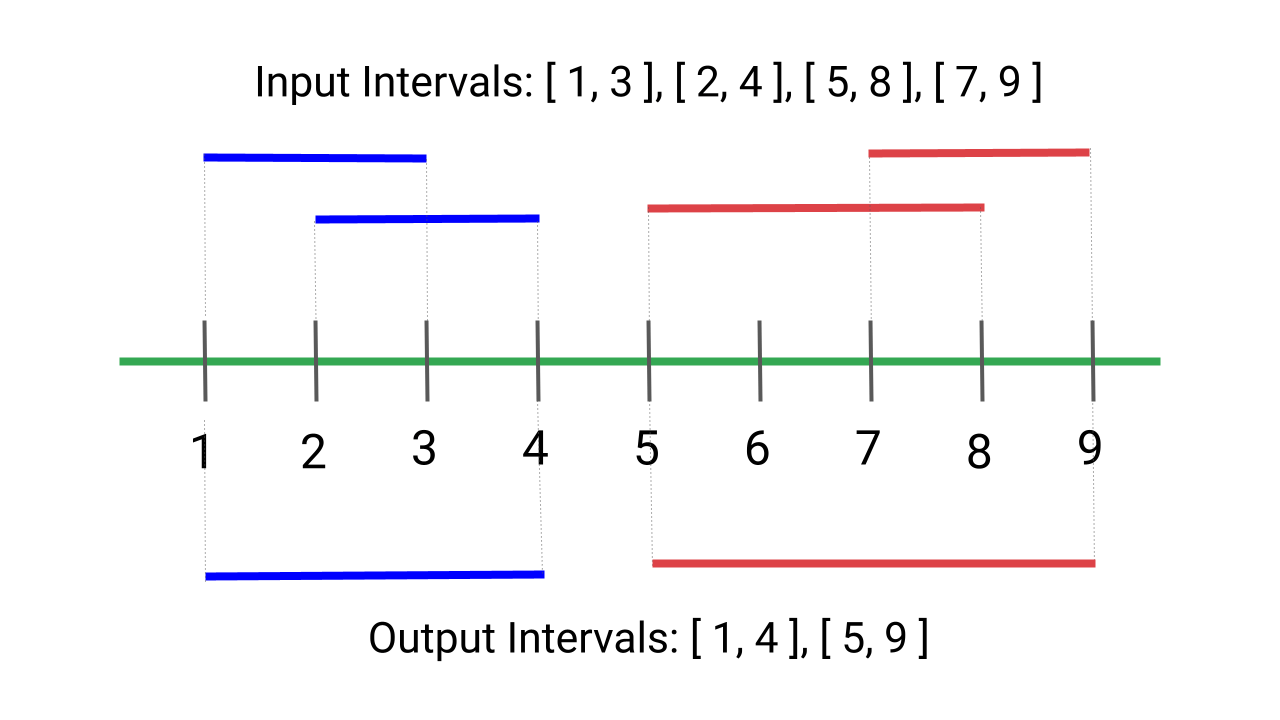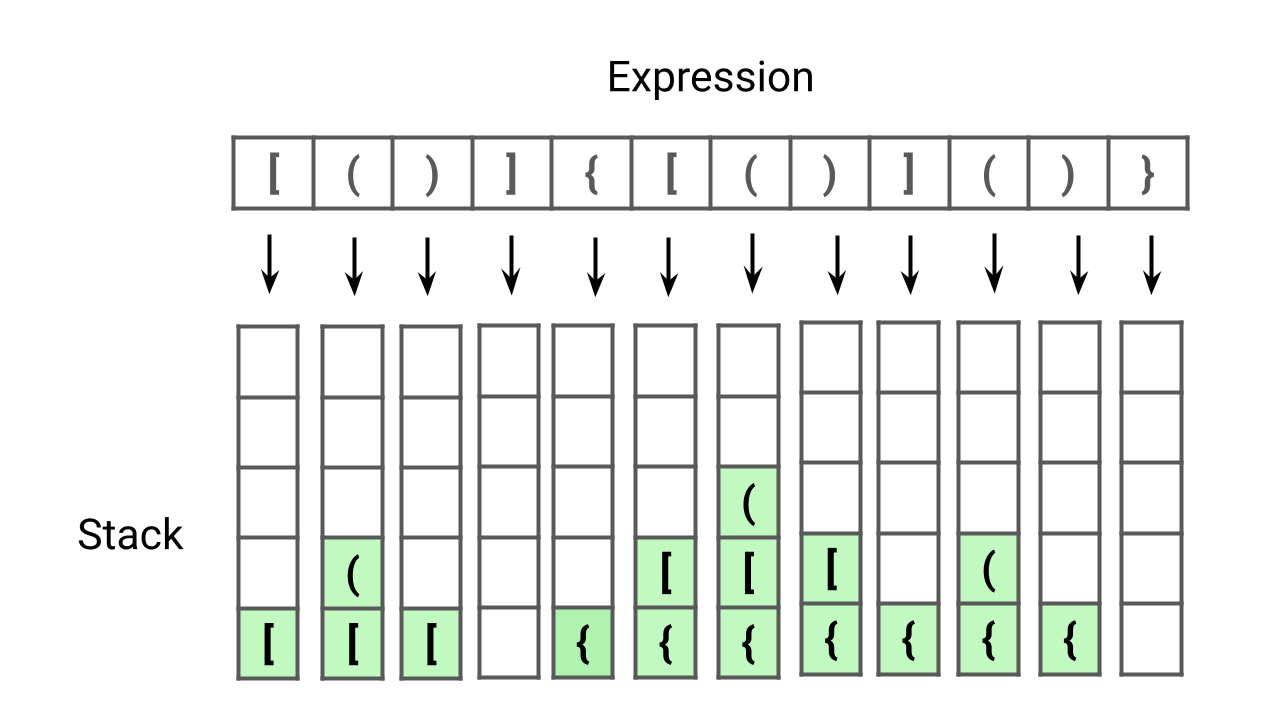# stackFlood Fill Algorithm

The flood fill problem is a way to fill a region of connected pixels with a new colour, starting from a given pixel in an image. You are given an image represented by an m x n grid of integers, where the image[i] represents the pixel value at position (i, j). You are also given the coordinates of a starting pixel (x, y) and a new colour to use for the flood fill operation.Min Stack Problem

Design and implement a stack that supports the push, pop, top, and retrieval of the minimum element in constant time. In other words, our goal is to implement the MinStack class, which supports push, pop, top, and getMin operations with O(1) time complexity. Note: The pop, top, and getMin operations will always be called on non-empty stacks.Merge Overlapping Intervals

Given an array of intervals where the start and end points of each interval are provided, write a program to merge all overlapping intervals and return an array of non-overlapping intervals. In other words, the output should be an array of mutually exclusive intervals. Note: This is an excellent problem to learn problem solving using sorting.Valid Parentheses Expression

Given an expression string containing opening and closing parentheses, write a program to check if the expression is balanced or not. An expression is considered balanced if each opening parenthesis is closed by the same type of closing parenthesis in the exact same order. This problem is excellent for learning problem-solving using stacks.Iterative Preorder, Inorder and Postorder Traversal Using Stack

We can easily implement recursive binary tree traversals (preorder, inorder, and postorder) iteratively using a stack. We need to understand the flow of recursive calls in DFS traversal and mimic what the compiler does in the background. So, we need to follow a similar process and use our own stack to simulate the recursive binary tree traversal using iteration or without using recursion.Real-life Applications of Stack Data Structure

Stack helps us in easy access and removal of the most recently added element. So there are several applications of the stack in data structures and algorithms. Some popular applications are: 1) Back and forward buttons in the browser 2) UNDO/REDO functionality in text editors 3) Memory management 4) Delimiter checking 5) Implementing recursion 6) Expression conversion and evaluation, etc.Stack Data Structure Operations and Implementation

The stack data structure is a type of collection that follows the Last In, First Out (LIFO) principle, meaning that the last item added to the stack will be the first one to be removed.Stack is a linear data structure that supports two primary operations: push and pop. When we add an element to the top, it is called a push operation. When we remove an element from the top, it is called a pop operation.Implement Stack using Queues

Write a program to implement a stack using queues. We must use queue operations like enqueue, dequeue, front, size to implement stack operations like push, pop, and top. We have discussed three approaches: 1) Using two queues: O(n) pop and O(1) push 2) Using two queues: O(1) pop and O(n) push 3) Using one queue: O(1) pop and O(n) push.Implement Queue Using Stacks

Write a program to implement queue using stack. We should use stack operations like push, pop, top, size, and isEmpty for implementing queue operations like enqueue, dequeue, and front. In this blog, we have discussed two approaches for implementing queue using two stacks: 1) Dequeue O(1) and Enqueue O(n) 2) Enqueue O(1) and Dequeue O(1).Sort a Stack

Given a stack, write a program to sort a stack in ascending order. We are not allowed to make assumptions about how the stack is implemented. The only functions to be used are push(s, x), pop(s), top(s), isEmpty(s). In this blog, we have discussed two approaches: 1) Sorting stack using temporary stack 2) Sorting stack using recursion.Find Next Greater Element

Given an array, find the next greater element for every element in the array (NGE). The next greatest element for an element is the first largest element on the right side. For elements for which no next largest element exists, consider the next greater element as -1. We have discussed two stack-based solutions: 1) Traversing from left to right, 2) Traversing from right to left.Trapping Rain Water

Given n non-negative integers representing an elevation map where the width of each bar is 1. Write a program to compute how much water it can trap after raining. This is a famous interview problem to learn time and space complexity optimization using various approaches. Two pointers approach provides an efficient solution using O(n) time and O(1) space.